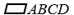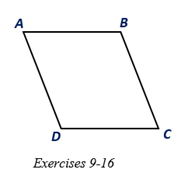Chapter 4.1, Problem 14E### Elementary Geometry for College St...

6th Edition
Daniel C. Alexander + 1 other
ISBN: 9781285195698

#### Solutions

Chapter
Section### Elementary Geometry for College St...

6th Edition
Daniel C. Alexander + 1 other
ISBN: 9781285195698
Textbook Problem
1 views

# Given that m ∠ A = 2 x + y , m ∠ B = 2 x + 3 y - 20 , and m ∠ C = 3 x - y + 16 , find the measure of each angle of.To determine

To find:

The measure of each angle of ABCD.

Explanation

Given:

Given that mA=2x+y, mB=2x+3y-20, and mC=3x-y+16, and the ABCD as shown below.

Corollary:

1. The opposite angles of a parallelogram are congruent.

2. Two consecutive angles of a parallelogram are supplementary.

Calculation:

In the given parallelogram ABCD, given that mA=2x+y, mB=2x+3y-20, and

mC=3x-y+16.

As per the corollary that the two consecutive angles of a parallelogram are supplementary, mA+mB=180

To find the value of x, substitute mA=2x+y, mB=2x+3y-20 in

mA+mB=180

2x+y+2x+3y-20=180

4x+4y-20=180

4x+4y=180+20

4x+4y=200

x+y=50 … (1)

As per the corollary that the opposite angles of a parallelogram are congruent, mA=mC

2x+y=3x-y+16

2x+y-3x+y=16

### Still sussing out bartleby?

Check out a sample textbook solution.

See a sample solution

#### The Solution to Your Study Problems

Bartleby provides explanations to thousands of textbook problems written by our experts, many with advanced degrees!

Get Started

#### In problems 24-26, find the intercepts and graph. 25.

Mathematical Applications for the Management, Life, and Social Sciences

#### Solve for x: ln(e + x) = 1. a) 0 b) 1 c) e d) ee e

Study Guide for Stewart's Single Variable Calculus: Early Transcendentals, 8th

#### True or False: converges if and only if .

Study Guide for Stewart's Multivariable Calculus, 8th

#### Explain the difference between passive and active deception.

Research Methods for the Behavioral Sciences (MindTap Course List)